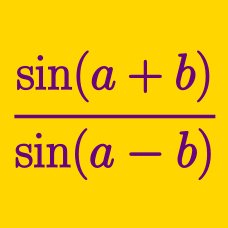Geometry

# Inverse Trigonometric Identities

Given that $\cos ( \arcsin \frac {12}{13} - \arctan \frac {16}{12} ) = \frac {a }{b}$ where $a$ and $b$ are positive coprime integers, what is the value of $a+b$?

Let $x = \sin\left(\sin^{-1} \left(\frac{3}{5}\right) + \tan^{-1} (2)\right)$. $x$ can be written in the form $\frac{a\sqrt{b}}{c}$, where $a, b$ and $c$ are positive integers, $a$ and $c$ are coprime and $b$ is not divisible by the square of any prime. What is the value of $a+b+c$?

Details and assumptions

$\sin^{-1}$ and $\tan^{-1}$ represent the inverse of the $\sin$ and $\tan$ function, and not the reciprocal.

Which of the following is equal to

$\arccos ( - x) ?$

If $x \in (0, \pi )$, which of the following is equal to

$\arctan ( \cot x) ?$

$\cos^{-1} \frac{a}{b} = 2 \tan^{-1} \frac{ \sqrt{72}} {12 },$ where $a$ and $b$ are positive, coprime integers. What is $a+b$?

×